Next: Conclusions Up: Calculating the Schiff moment Previous: Odd- Nuclei and Schiff

## Laboratory Schiff Moment of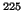Ra

We turn finally to results inRa itself. For SkO', our HFODD calculations yield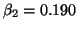,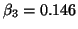, and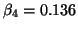for the usual first order approximation to the deformation parameters determined from mass multipole moments [Ring and Schuck(1980)]. The laboratory Schiff moment, Eq. (6), is proportional to the product of the intrinsic Schiff moment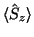and the expectation value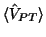. Table I shows the intrinsic moments and the expectation values of the 6 operators that enter the zero-range approximation to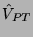in Eq. (10). Before commenting on the entries, we mention what is in each of the forces and calculations.

For all the forces, terms in the functional that are proportional to Laplacians of spin densities (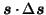) and density-dependent spin-spin terms (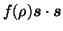), cf. Ref. [Dobaczewski and Dudek(1995),Bender et al.(2002)Bender, Dobaczewski, Engel, and Nazarewicz], which enter through the T-odd part of the Skyrme functional, have been turned off. For the first three lines in Table I [forces labeled with (0)], the spin-spin terms have also been turned off, so that the only nonzero terms in the T-odd functional (as noted above) are those required by gauge invariance. For the fourth line [SkO'(--)], all T-odd terms in the functional have been turned off, so that aside from the self-consistency in the wave functions the calculation resembles one with a phenomenological (non-self-consistent) potential, for which T-odd mean-fields are never considered. We include this result so that we can distinguish the role played by core polarization. The results in the line labeled SkO' include the time-odd channels, adjusted as discussed above [Bender et al.(2002)Bender, Dobaczewski, Engel, and Nazarewicz]. This is the force in which we have the most confidence. The last entry is the result of Ref. [Spevak et al.(1997)Spevak, Auerbach, and Flambaum], with the implicit assumption that the neutron and proton densities are proportional.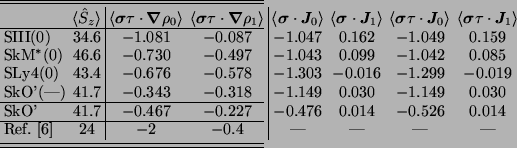In our calculations, the intrinsic Schiff moments are close to one another, and all are less than twice the estimate of Ref. [Spevak et al.(1997)Spevak, Auerbach, and Flambaum]. The agreement reflects the collective nature of these intrinsic moments. But the matrix elements of, the other ingredient in Eq. (6) for the laboratory Schiff moment, are a bit more delicate. Our results show the exchange terms on the right side of the table to be comparable to the direct terms, a result that is surprising because for a spin-saturated core (or in the particle-rotor model) the exchange terms vanish exactly. We think, however, that the ratio of exchange to direct terms would become small were the finite range of the interaction reintroduced and short-range NN correlations inserted.

Though unable to include either effect here, we did so in a Nilsson model forRa. We took nucleons there to occupy independent single-particle levels generated by a deformed oscillator potential with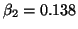,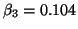, and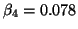, values taken from Ref. [Spevak et al.(1997)Spevak, Auerbach, and Flambaum]. We then evaluated the ground-state expectation value of the full two-body interaction, with and without the zero-range approximation (and in the latter case, with short-range correlations included à la Ref. [Miller and Spencer(1976)]). In this simple model, the valence nucleon carries all the spin, and only the neutron-proton and neutron-neutron parts ofcontribute. The direct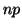term shrank by a factor of 1.5, while the corresponding exchange term shrank by a factor of 1400 (both independently of the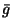's in Eq. (4), it turns out) when the range of the interaction was set to its proper value. The results in the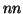channel were less dramatic: the direct part again shrank by 1.5 and the exchange part by a factor of 5. When we moved the valence neutron to higher orbits, these numbers changed some -- the direct terms sometimes were not suppressed at all and other times shrank by factors of up to 6, but the ratios of the exchange to direct contributions almost always ended up small. Similar behavior was found for parity-violating forces in Ref. [Adelberger and Haxton(1985)], where it was traced in part to the different average momenta carried by the pion in direct and exchange graphs. So that we can compare our results with those of Ref. [Spevak et al.(1997)Spevak, Auerbach, and Flambaum], we will neglect the exchange terms from now on, though we caution that this step should eventually be justified more rigorously, e.g., by actually calculating them with the finite-range force in the full mean-field model. The reduction we see in the direct terms is in line with the results of Ref. [Griffiths and Vogel(1991)], though we find it more variable.

Though we cannot yet be more quantitative about finite-range effects, we do quantify the core polarization in Table I. For the first three lines of the table, where the forces are labeled (0), the spin-spin terms are absent from the energy functional, and the protons in the core develop only a tiny spin density from the T-odd terms required by gauge invariance. For the fourth line, SkO'(--), all T-odd terms are absent and the protons can have no spin at all. This means that the operators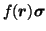and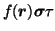have either the same or almost the same expectation value for any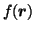so that columns 4 and 6 (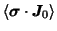and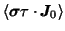) have identical or nearly identical entries for these forces, and so do columns 5 and 7 (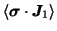and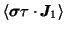). The fifth line of the table contains the effects of spin polarization, which are primarily to alter the neutron-spin density; the equalities between the columns are not badly broken, so the protons do not develop much spin. The same is true of the terms involving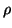, though that is not obvious from the table because we display only the two terms that appear in Eq. (10).

These near equalities and the probable irrelevance of the exchange terms when the finite range is taken into account imply that only the quantities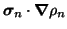and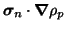are ultimately important. We display them in Table II. Except for SIII, the neutron-density distribution affects the matrix element much more than the that of protons. By comparing the fourth and fifth lines, however, we see that spin correlations increase the role of the protons, while reducing that of the neutrons slightly. Thus, while the spin-spin interactions do not cause the protons to develop much net spin, they do correlate the neutron spin with the proton density.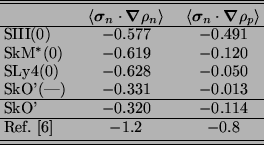There is not too much scatter in our results. The entries in the second column (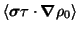) of Table I differ by factors of two or three, and the entries in the third (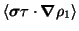) by a little more, though they are all smaller than those in the second column (which is not surprising -- the third column subtracts the neutron and proton densities while the second adds them). In the neutron-proton scheme (table II) all of our numbers are smaller than those of Ref. [Spevak et al.(1997)Spevak, Auerbach, and Flambaum], a result that was anticipated in Ref. [Engel et al.(1999)Engel, Friar, and Hayes]. The difference from the earlier estimate for the larger matrix elements ranges from factors of two to four, though the isovector combination -- the third column in table I -- is sometimes actually enhanced a little.

What, at last, have we to say about the real laboratory Schiff moment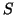? The lab moment is given by the product of the matrix elements just discussed, the intrinsic Schiff moments, and the unknown coefficients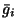. Our intrinsic Schiff moments are about 1.5 times larger than those of Ref. [Spevak et al.(1997)Spevak, Auerbach, and Flambaum], while ourmatrix elements, in the zero-range approximation, are smaller than theirs, usually by a somewhat larger amount. Overall, our lab moments will usually be smaller by factors between about 1.5 and 3 than the estimates of Ref. [Spevak et al.(1997)Spevak, Auerbach, and Flambaum] (an exception can occur if for some reason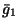is considerably less than the other two coefficients).

How big are our moments compared to that of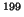Hg? The most comprehensive calculation in that nucleus, which appeared very recently [Dmitriev and Sen'kov()], improved on the work of Ref. [Flambaum et al.(1986)Flambaum, Khriplovich, and Sushkov] by including the effects of the residual strong interaction and the full finite-range form for. The new results are smaller than that of ref. [Flambaum et al.(1986)Flambaum, Khriplovich, and Sushkov], only slightly so for the isovector part of, but by a considerably amount in the isoscalar and isotensor channels. The authors write their results in terms of the pion-nucleon couplings as The very small coefficient of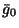forHg in Eq. (11) has significant consequences for the limit on the QCD T-violating paramter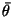that can be inferred from the experimental limit in Ref. [Romalis et al.(2001)Romalis, Griffith, Jacobs, and Fortson]. See Ref. [Herczeg(1988b)].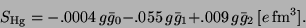(8)

Our result for radium, with the zero-range approximation and exchange terms neglected, translates to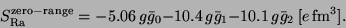(9)

If the three's are comparable, therefore, our Schiff moment is several hundred times larger than that of Ref. [Dmitriev and Sen'kov()], in part because the isoscalar and isotensor interactions are more effective in Ra than in Hg. [Ifis larger than the other two couplings, as in left-right symmetric models [Herczeg(1988b)], our result is less than 200 times bigger than the latest one inHg. The very small coefficient offorHg in Eq. (11), by the way, has significant consequences [Herczeg(1988b)] for the limit on the QCD T-violating parameterthat can be inferred from the experimental limit in Ref. [Romalis et al.(2001)Romalis, Griffith, Jacobs, and Fortson].] Accepting the work of Ref. [Spevak et al.(1997)Spevak, Auerbach, and Flambaum] on atomic physics in Ra and Hg, the enhancement of the atomic EDM ofRa is about about twice that of the Schiff moment, i.e. potentially more than 1000. We again caution, however, that we have yet to include the full finite-range version ofand that our preliminary investigations suggest that doing so will decrease our Schiff moment at least a little. Ironically, Ref. [Dmitriev and Sen'kov()] finds that including the finite range actually increases the matrix element inHg, though only slightly.

We hope to make other improvements in our calculation as well. Projection onto states of good parity will change the results a bit, as will angular-momentum projection. Our conclusions about the size of spin-polarization corrections could be modified by two terms in the Skyrme functional we've set to zero, or by a better determined value of the Landau parameter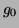.Next: Conclusions Up: Calculating the Schiff moment Previous: Odd- Nuclei and Schiff
Jacek Dobaczewski 2003-04-24